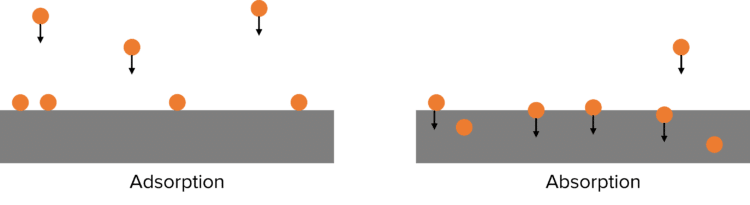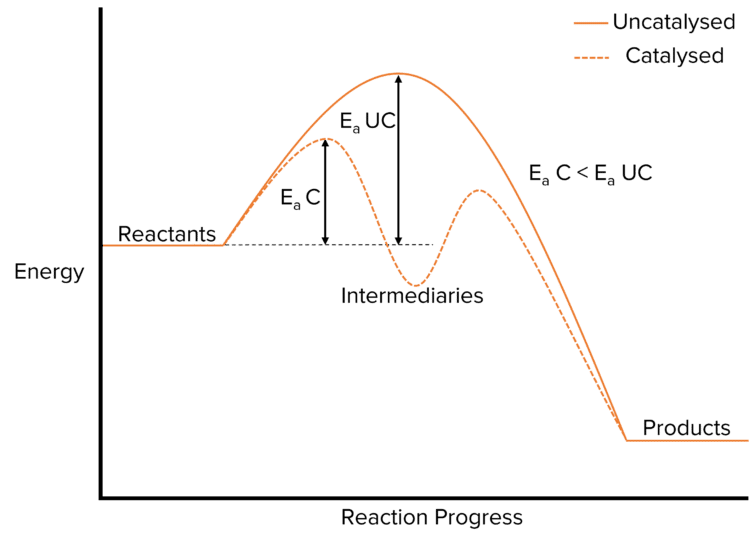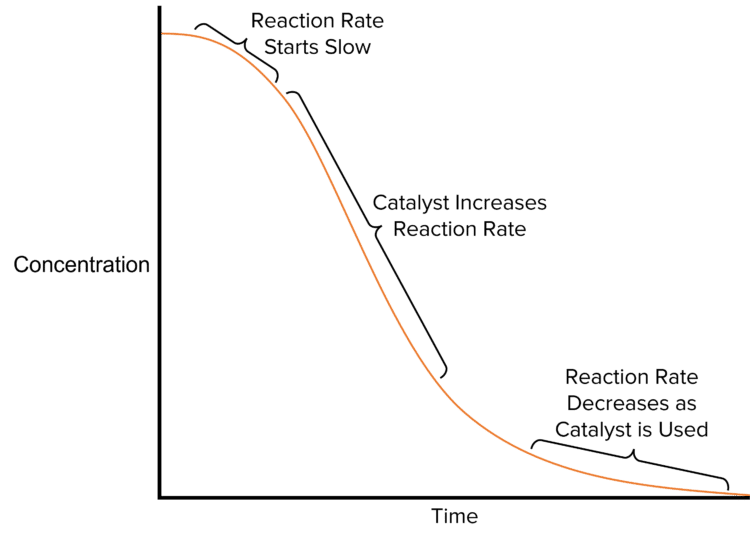# Catalyst

A LevelAQA

## Catalysts

Catalysts are substances that speed up the rate of reaction by providing an alternative route with a lower activation energy. They can be heterogeneous or homogeneous. Transition metals are good catalysts as they exist in variable oxidation states and can therefore transfer electrons to speed up reactions.

## Heterogeneous Catalysts

Heterogeneous catalysts are catalysts that are in a different phase from the reactants and they are usually solid. They work by adsorbing reactants at the active sites on their surfaces.

The effectiveness of a heterogeneous catalyst depends upon the strength at which it can adsorb reactants. When chemicals are adsorbed, they become stuck to the surface of a material.This is in contrast to absorption where molecules penetrate below the surface of a material. If adsorption is too strong, then the products formed cannot be released from the surface of the catalyst. If the adsorption is too weak, the reactants cannot adsorb to surface of the catalyst.

Increasing the surface area of a catalyst can also increase effectiveness. To achieve this, a support medium can be used to maximise the surface area of the catalyst and minimise cost. For example, in catalytic converters, which are used to convert toxic gases to less harmful gases, a platinum catalyst is placed on a ceramic support medium to increase the surface area and effect of the catalyst.

Catalytic Poisoning
When impurities are in the reaction mixture they can bind to the catalyst surface and block the active sites.

This reduces the catalyst’s efficiency and increases the reaction’s cost. The catalyst will also need to be replaced more frequently because of the blocked active sites.

In the Haber process, sulfur impurities can bind to the catalyst and poison it.

A LevelAQA

## Examples of Heterogenous Catalysis

Contact Process

In the contact process, Vanadium (V) oxide is used as a catalyst to convert sulfur dioxide into sulfur trioxide. The process involves 2 intermediate steps where the vanadium is reduced.

Step 1:$\text{V}_2\text{O}_5 + \text{SO}_2 \rarr \text{V}_2\text{O}_4 + \text{SO}_3$
Step 2:         $\text{V}_2\text{O}_4 + \frac{1}{2}\text{O}_2 \rarr \text{V}_2\text{O}_5$

Overall Reaction: $\text{SO}_2 + \frac{1}{2}\text{O}_2 \rarr \text{SO}_3$

Haber Process

In the Haber process (the process used to make ammonia), iron is used as the heterogeneous catalyst. It speeds up the reaction between hydrogen and nitrogen gas.

Haber Process:$\text{N}_2 + 3\text{H}_2 \rarr 2\text{NH}_3$

A LevelAQA

## Homogenous Catalysts

A homogeneous catalyst is a catalyst that is in the same phase as the reactants. Since the reactants are in the same phase, an intermediate species is needed for the reaction to take place.

Reactions with homogeneous catalysts have 2 steps, this results in a lower activation energy than if the reaction had taken place in one step.One important reaction to be aware of is the reaction between iodide ions and peroxodisulfate because this reaction requires a homogenous catalyst in order to take place.

The reaction between iodine ions $\left(\text{I}^-\right)$ and peroxodisulfate $\left(\text{S}_2\text{O}_8^{2-}\right)$ is very slow as both ions are negatively charged and therefore repel.

Overall Reaction: $\text{S}_2\text{O}_8^{2-} + 2\text{I}^- \rarr \text{I}_2 + 2\text{SO}_4^{2-}$

$\text{Fe}^{2-}$ is used to speed up this reaction; as it is a positive ion, there is no repulsion and it can attract the negative ions in the reaction.

When the reaction is catalysed by $\text{Fe}^{2+}$, the following reactions take place.

Step 1: $\text{S}_2\text{O}_8^{2-} + 2\text{Fe}^{2+} \rarr 2\text{Fe}^{3+} + 2\text{SO}_4{2-}$
Step 2:                                           $2\text{Fe}^{3+} + 2\text{I}^- \rarr \text{I}_2 + 2\text{Fe}^{2+}$

A LevelAQA

## Autocatalysis

Autocatalysis occurs when one of the reaction products is a catalyst for the reaction. As the reaction progresses, it speeds up due to the larger amount of catalyst.An example of autocatalysis is the reaction of manganate and ethanedioate ions. In this reaction, $\text{MnO}_4^-$ ions form $\text{Mn}^{2+}$ ions. These ions then go on to react with $\text{C}_2\text{O}_4^{2-}$ to complete the reaction.

Step 1: $\text{MnO}_4^- + 4\text{Mn}^{2+} + 8\text{H}^+ \rarr 5\text{Mn}^{3+} + 4\text{H}_2\text{O}$

Step 2:                      $2\text{Mn}^{3+} + \text{C}_2\text{O}_4^{2-} \rarr 2\text{Mn}^{2+} + 2\text{CO}_2$

Overall Reaction: $2\text{MnO}_4^- + 16\text{H}^+ + 5\text{C}_2\text{O}_4^{2-} \rarr 2\text{Mn}^{2+} + 8\text{H}_2\text{O} + 10\text{CO}_2$

A LevelAQA

## Catalyst Example Questions

a.) Both ions are negative and so will repel each other, preventing collisions.

b.) $\text{Fe}^{2+}$ is a positive ion so can attract the negative ions in the reaction.

a.) A catalyst that is in a different phase or state to the reactants.

b.)

$\text{V}_2\text{O}_5 + \text{SO}_2 \rarr \text{V}_2\text{O}_4 + \text{SO}_3$

$\text{V}_2\text{O}_4 + \frac{1}{2}\text{O}_2 \rarr \text{V}_2\text{O}_5$

a.) The product of the reaction acts as a catalyst.

b.)

$\text{MnO}_4^- + 4\text{Mn}^{2+} + 8\text{H}^+ \rarr 5\text{Mn}^{3+} + 4\text{H}_2\text{O}$

$2\text{Mn}^{3+} + \text{C}_2\text{O}_4^{2-} \rarr 2\text{Mn}^{2+} + 2\text{CO}_2$

The impurities block the active sites (OR deactivate the catalyst).

## You May Also Like...### MME Learning Portal

Online exams, practice questions and revision videos for every GCSE level 9-1 topic! No fees, no trial period, just totally free access to the UK’s best GCSE maths revision platform.

£0.00